### IMO Shortlist 1988 problem 10

Kvaliteta:
Avg: 0,0
Težina:
Avg: 0,0
Let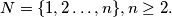$N = \{1,2 \ldots, n\}, n \geq 2.$ A collection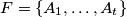$F = \{A_1, \ldots, A_t\}$ of subsets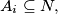$A_i \subseteq N,$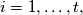$i = 1, \ldots, t,$ is said to be separating, if for every pair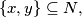$\{x,y\} \subseteq N,$ there is a set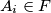$A_i \in F$ so that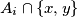$A_i \cap \{x,y\}$ contains just one element.$F$ is said to be covering, if every element of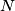$N$ is contained in at least one set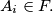$A_i \in F.$ What is the smallest value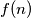$f(n)$ of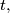$t,$ so there is a set$F = \{A_1, \ldots, A_t\}$ which is simultaneously separating and covering?
Izvor: Međunarodna matematička olimpijada, shortlist 1988